EnchantedLearning.com is a user-supported site.
As a bonus, site members have access to a banner-ad-free version of the site, with print-friendly pages.

 +, - EnchantedLearning.comMath Dictionary x, ÷
 A B C D E F G H I J K L M N O P Q R S T U V W X Y ZR is for ...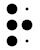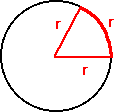radianA radian is a unit of angular measure that is equal to the angle subtended at the center of a circle by an arc equal in length to the radius of the circle. A radian is about 57.2957° (1 degree = 0.0174532 radians). There are 2π radians in 360°.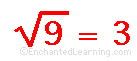radicalA radical is a symbol √ that is used to indicate the square root or nth root of a number.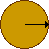radiusThe radius is the distance from the center of a circle (or a sphere) to the edge.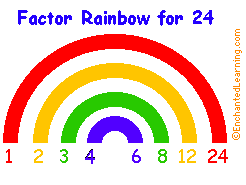factor rainbowA factor rainbow is a rainbow-shaped diagram that factors of a number in pairs. In the example above, the factors of 24 are: 1*24, 2*12, 3*8, and 4*6. For a prime number (for example, 3 or 15), there will be only one pair of factors, one and the number itself. For a square number (for example, 9 or 16), there will be a single factor in the middle of the rainbow.
 1/2ratioA ratio is the relationship between two numbers, denoted by division. For example, if the ratio of boys to girls at a school is 4 to 5, this can be written as 4/5 or 4:5. rational numberA rational number is a number that can be expressed in the form a/b, where a and b are integers and b≠0. For example, 0, 1/2, and -41 are rational numbers. Each rational number corresponds to a unique point on the number line (but most points on the number line do not correspond to a rational number -- they correspond to an irrational number).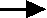rayA ray (also called a half line) is the portion of a straight line that extends infinitely far in one direction from a point. real numberReal numbers are all rational and irrational numbers (but not the imaginary numbers). The real numbers represent all the points on the number line.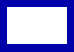rectangleA rectangle is a four-sided figure (a quadrilateral) whose sides are at right angles to each other. A square is a type of rectangle whose four sides are all the same length.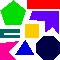regular polygonA regular polygon is a polygon (a polygon is a many-sided closed figure with straight edges) whose sides are all the same length and whose interior angles are all the same (like an equilateral triangle or a square).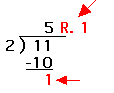remainderThe remainder is the part part of the answer in long division that does not go evenly into the divisor. The dividend divided by the divisor is the quotient plus the remainder. rhomboidA rhomboid is a parallelogram with oblique angles and adjacent sides have different lengths.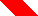rhombusA rhombus is a parallelogram with equal-length sides.right angleA right angle looks like the corner of a square; it extends ninety degrees. Each of the interior angles of a square is a right angle.right triangleA right triangle has one angle that is a right angle (extending 90 degrees).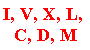Roman numeralsThousands of years ago, in ancient Rome, the Romans used a system of numbers that we call Roman numerals. In this system, I=1, V=5, X=10, L=50, C=100, D=500, and M=1,000. Roman numerals express numbers as sums and differences. For example, 6 is VI (five + one), but nine is IX (ten - one). In general, when a smaller Roman numeral follows a larger numeral, you add the numbers (for example, XII is ten + one + one = twelve). When a larger numeral follows a smaller one, you subtract the numbers (for example, IV is five - one = four, and XL is 50 - 10 = 40).rootAn nth root of a number is a number that, when multiplied by itself n times, results in that number. For example, the number 4 is a square root of 16 because 4 x 4 equals 16. The number 2 is a cube root of 8 because 2 x 2 x 2 equals 8.roundRound things are curved. A circle is round.
Number Nearest ten Nearest hundred Nearest thousand
368 370 400 0
1,234 1,230 1,200 1,000
89,355 89,360 89,400 89,000

rounding

When a number is rounded (or rounded off), it is approximated by eliminating the least significant digits. Whole numbers can be rounded to the tens place, hundreds place, thousands place, and so on. Decimals can also be rounded, estimating the number to the nearest tenth, hundredth, thousandth, and so on. Rounding is used to make a number easier to work with. For example, if you know that there are 496 students in your school, you can say that there are approximately 500 students in your school.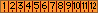ruler

A ruler is a long, straight object that is used for measuring distances or drawing straight lines.

 +, - EnchantedLearning.comMath Dictionary x, ÷
 A B C D E F G H I J K L M N O P Q R S T U V W X Y Z
 Number Line Fractions Decimals Graphs Measurement Rounding

Click on an underlined word for more information on that subject. If the math term you are looking for is not in the dictionary, please e-mail us.

Enchanted Learning®
Over 35,000 Web Pages
Sample Pages for Prospective Subscribers, or click below

 Overview of Site What's New Enchanted Learning Home Monthly Activity Calendar Books to Print Site Index K-3 Crafts K-3 Themes Little ExplorersPicture dictionary PreK/K Activities Rebus Rhymes Stories Writing Cloze Activities Essay Topics Newspaper Writing Activities Parts of Speech Fiction The Test of Time Biology Animal Printouts Biology Label Printouts Biomes Birds Butterflies Dinosaurs Food Chain Human Anatomy Mammals Plants Rainforests Sharks Whales Physical Sciences: K-12 Astronomy The Earth Geology Hurricanes Landforms Oceans Tsunami Volcano Languages Dutch French German Italian Japanese (Romaji) Portuguese Spanish Swedish Geography/History Explorers Flags Geography Inventors US History Other Topics Art and Artists Calendars College Finder Crafts Graphic Organizers Label Me! Printouts Math Music Word Wheels

## Enchanted Learning Search

 Search the Enchanted Learning website for: